﻿ Board Paper of Class 10 2022 Mathematics (Basic) Term 1 Set 4 Solutions | Aasoka

# Board Paper of Class 10 2022 Mathematics (Basic) Term 1 Set 4 - Solutions

#### General Instructions:

1. This question paper contains 50 questions out of which 40 questions are to be attempted. All questions carry equal marks.
2. This question paper contains three Sections: A, B, and C.
3. Section A has 20 questions. Attempt any 16 questions from Q.No. 1 to 20.
4. Section B has 20 questions. Attempt any 16 questions from Q.No. 21 to 40.
5. Section C contains two Case Studies containing 5 Questions in each case. Attempt any 4 questions from Q.No. 41 to 45 and 4 another from Q.No. 46 to 50.
6. There is only one correct option for every multiple choice question (MCQ). Marks will not be awarded for answering more than one option.
7. There is no negative marking.
(In this section, there are 20 questions. Any 16 are to be attempted).

## HCF of 92 and 152 is

1. 4
2. 19
3. 23
4. 27

Sol. (a);

The prime factorisation of 92 and 152 are: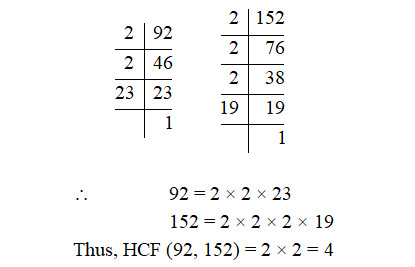## In $△$ABC, DE || BC, AD = 4 cm, DB = 6 cm and AE = 5 cm. The length of EC is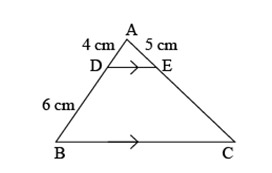1. 7 cm
2. 6.5 cm
3. 7.5 cm
4. 8 cm

Sol. (c); In $△$ABC, DE || BC by BPT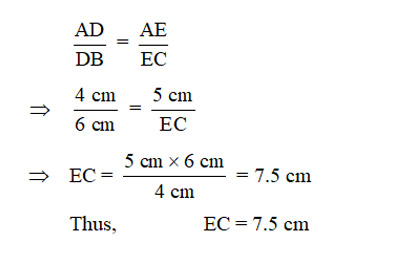## The value of k, for which the pair of linear equations x + y – 4 = 0, 2x + ky – 3 = 0 have no solution, is

1. 0
2. 2
3. 6
4. 8

Sol. (b); Since the given pair of linear equations has no solution.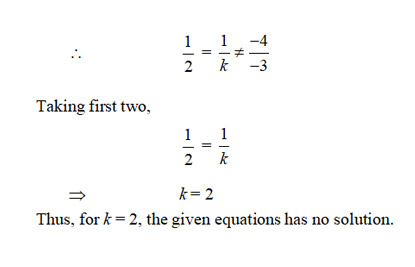## The value of (tan2 45° – cos2 60°) is

1. $\begin{array}{c}1\\ -\\ 2\end{array}$
2. $\begin{array}{c}1\\ -\\ 4\end{array}$
3. $\begin{array}{c}3\\ -\\ 2\end{array}$
4. $\begin{array}{c}3\\ -\\ 4\end{array}$

Sol. (d); We know that,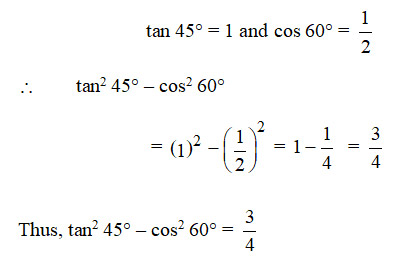## A point (x, 1) is equidistant from A(0, 0) and B(2, 0). The value of x is

1. 1
2. 0
3. 2
4. $\begin{array}{c}1\\ -\\ 2\end{array}$

Sol. (a); Let P(x, 1) be equidistant from A(0, 0) and B(2, 0)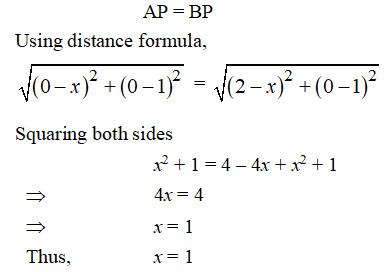## Two coins are tossed together. The probability of getting exactly one head is

1. $\begin{array}{c}1\\ -\\ 4\end{array}$
2. $\begin{array}{c}1\\ -\\ 2\end{array}$
3. $\begin{array}{c}3\\ -\\ 4\end{array}$
4. 1

Sol. (b); When two coins are tossed together, then the following outcomes are obtained

HH, HT, TH, TT

Exactly one head is obtained, if the events are:

HT, TH

Hence, P(exactly one head) $\begin{array}{cccc}& 2& & 1\\ =& -& =& -\\ & 4& & 2\end{array}$

## A circular arc of length 22 cm subtends an angle $\mathrm{\theta }$ at the centre of the circle of radius 21 cm. The value of $\mathrm{\theta }$ is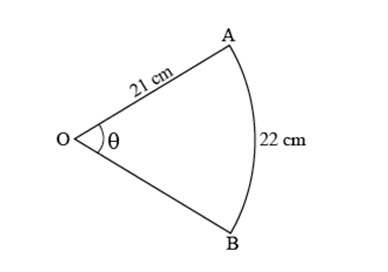1. 90°
2. 50°
3. 60°
4. 30°

(c); Length of arc (l) = 22 cm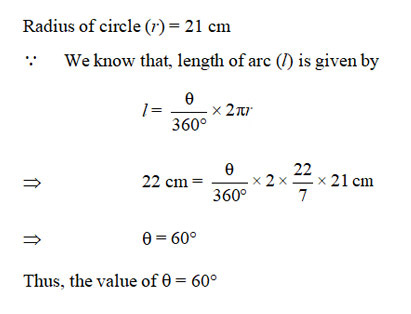## A quadratic polynomial having sum and product of its zeroes as 5 and 0 respectively, is

1. x2 + 5x
2. 2x(x – 5)
3. 5x2 - 1
4. x2 – 5x + 5

Sol. (b); Let $\alpha$ and $\beta$ be two roots of the given polynomial.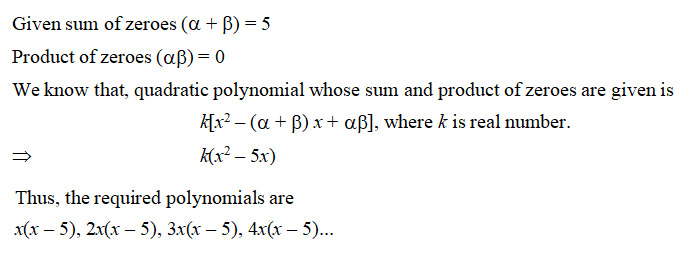## If P(E) = 0.65, then the value of P(not E) is

1. 1.65
2. 0.25
3. 0.65
4. 0.35

Sol. (d); We know that, sum of the probability of happening event (E) and not happening event ($\overline{E}$) is 1.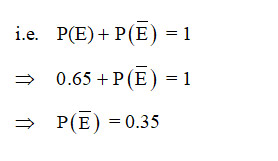## It is given that $△$DEF ~ $△$PQR. EF : QR = 3 : 2, then value of ar(DEF): ar(PQR) is

1. 4 : 9
2. 4 : 3
3. 9 : 2
4. 9 : 4

Sol. (d); Given that $△$DEF ~ $△$PQR and EF : QR = 3 : 2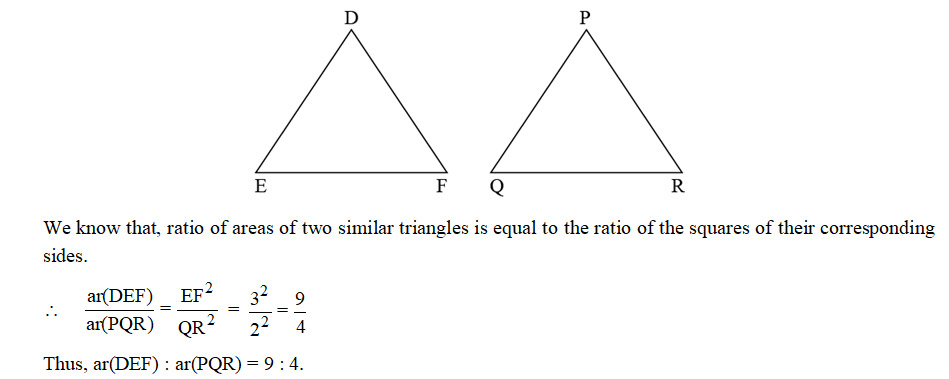## Zeroes of a quadratic polynomial x2 – 5x + 6 are

1. –5, 1
2. 5, 1
3. 2, 3
4. –2, –3

Sol. (c); Given quadratic polynomial is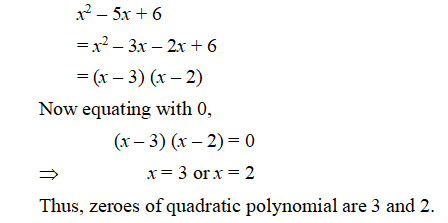## $\begin{array}{c}57\\ -\\ 300\end{array}$ is a

1. non-terminating and non-repeating decimal expansion.
2. terminating decimal expansion after 2 places of decimals.
3. terminating decimal expansion after 3 places of decimals.
4. non-terminating but repeated decimal expansion.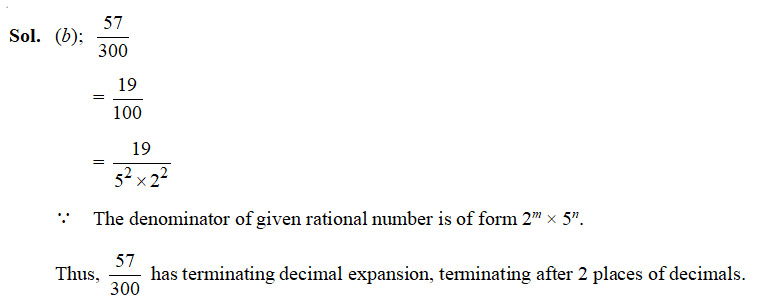## Perimeter of a rectangle whose length ($l$) is 4 cm more than twice its breadth (b) is 14 cm. The pair of linear equations representing the above information is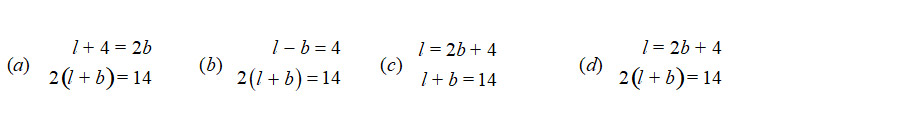Sol.(d); Let $l$ be the length and $b$ be the breadth.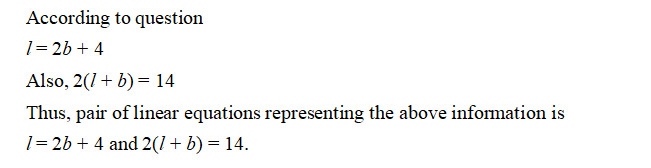##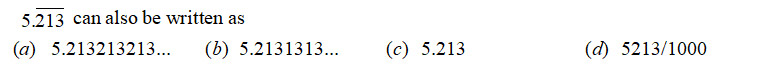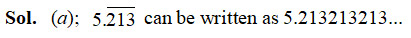## The ratio in which the point (4, 0) divides the line segment joining the points (4, 6) and (4, –8) is

1. 1 : 2
2. 3 : 4
3. 4 : 3
4. 1 : 1

Sol. (b); Let P(4, 0) divides the line segment joining the points A(4, 6) and B(4, –8) in the ratio k : 1.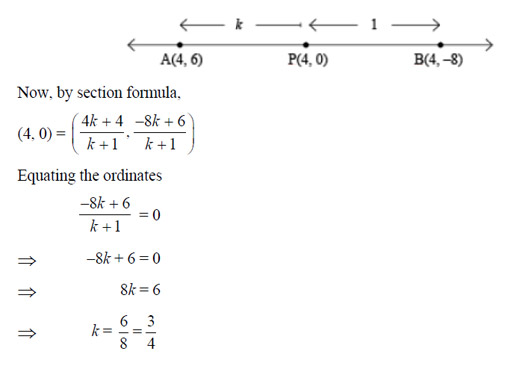##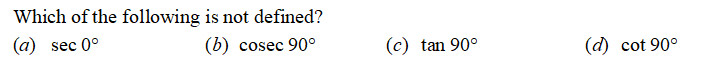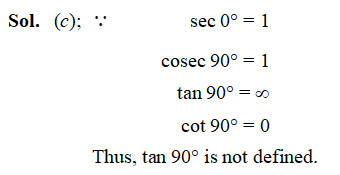## In the given figure, a circle is touching a semi-circle at C and its diameter AB at O. If AB = 28 cm, what is the radius of the inner circle?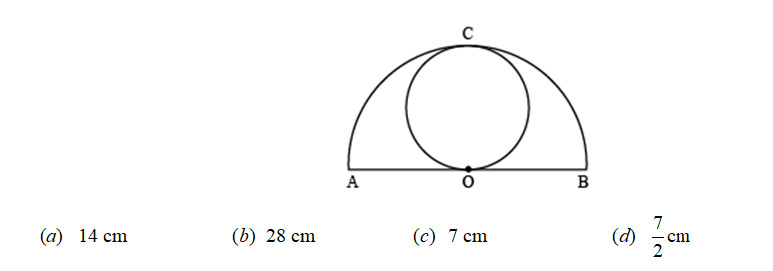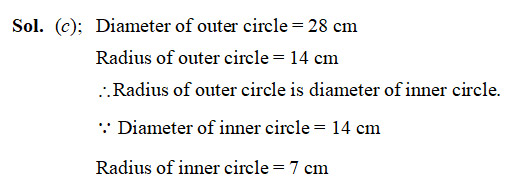## The vertices of a triangle OAB are O(0, 0), A(4, 0) and B(0, 6). The median AD is drawn on OB. The length AD is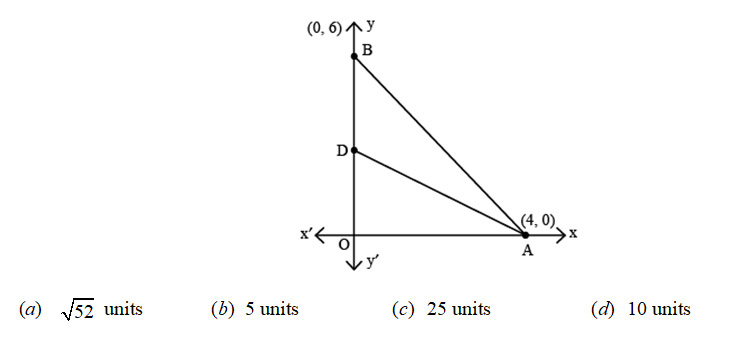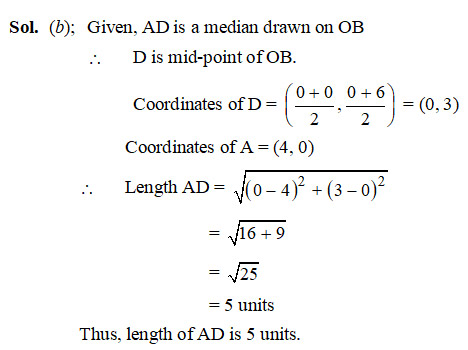##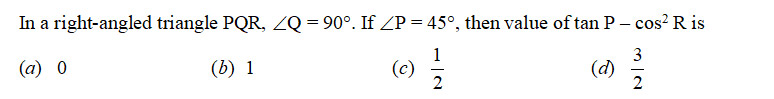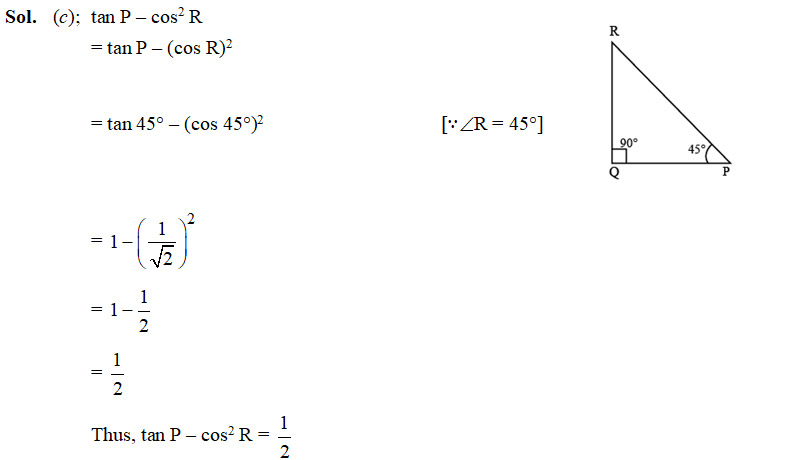##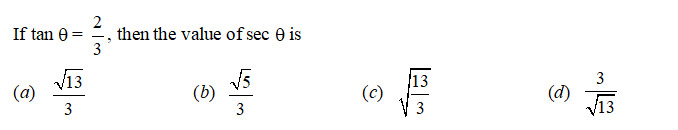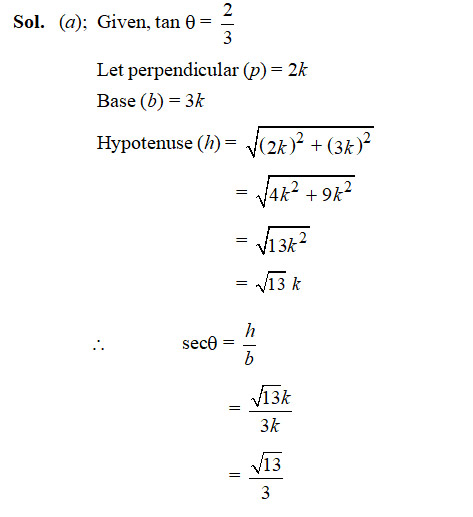(In this section, there are 20 questions. Any 16 are to be attempted).

## The perimeter of the sector of a circle of radius 14 cm and central angle 45° is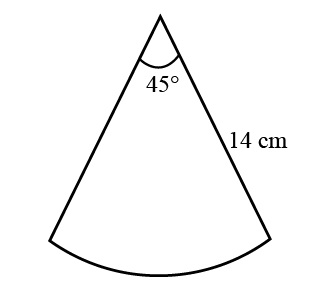1. 11 cm
2. 22 cm
3. 28 cm
4. 39 cm

Sol. (d);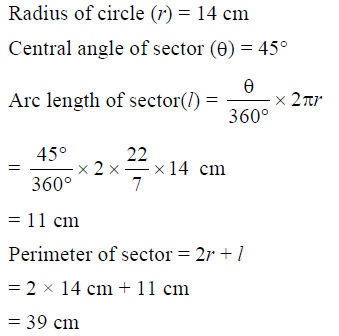## A bag contains 16 red balls, 8 green balls and 6 blue balls. One ball is drawn at random. The probability that it is blue ball is

1. 1/2
2. 1/5
3. 1/30
4. 5/6

Sol. (b);

Total number of balls in the bag = 16 + 8 + 6 = 30

Number of blue balls in the bag = 6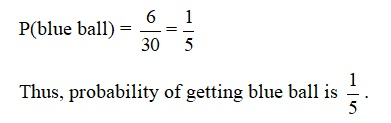## If sin$\mathrm{\theta }$ - cos$\mathrm{\theta }$ = 0, then the value of $\mathrm{\theta }$ is

1. 30°
2. 45°
3. 90°

Sol. (b); Given sin $\mathrm{\theta }$ - cos$\mathrm{\theta }$ = 0

Since, we know that sine and cosine have equal value at 45°.

Thus, $\mathrm{\theta }$ = 45°.

## The probability of happening of an event is 0.02. The probability of not happening of the event is

1. 0.02
2. 0.80
3. 0.98
4. 49/100

Sol. (c); We know that, sum of the probability of happening and not happening of an event is 1.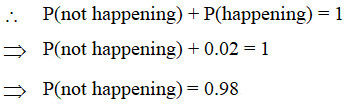## Two concentric circles are centred at O. The area of shaded region, if outer and inner radii are 14 cm and 7 cm respectively, is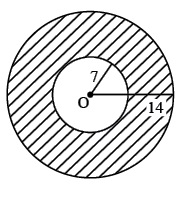1. 462 cm2
2. 154 cm2
3. 231 cm2
4. 308 cm2

(a); Outer radius (R) = 14 cm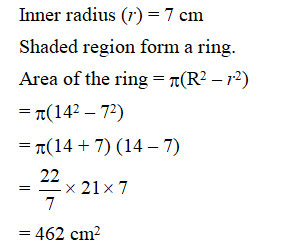##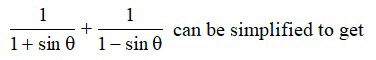1.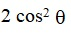2.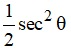3.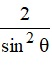4.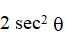Sol. (d);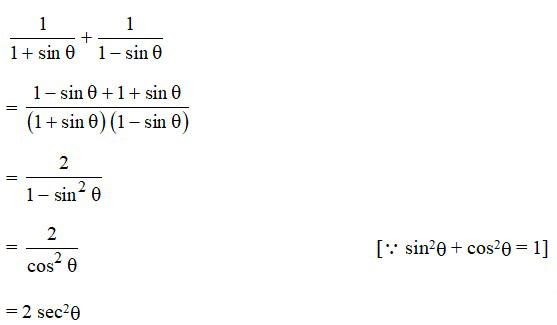## The origin divides the line segment AB joining the points A(1, -3) and B(-3, 9) in the ratio:

1. 3 : 1
2. 1 : 3
3. 2 : 3
4. 1 : 1

Sol. (b); Let origin divides AB in the ratio k : 1.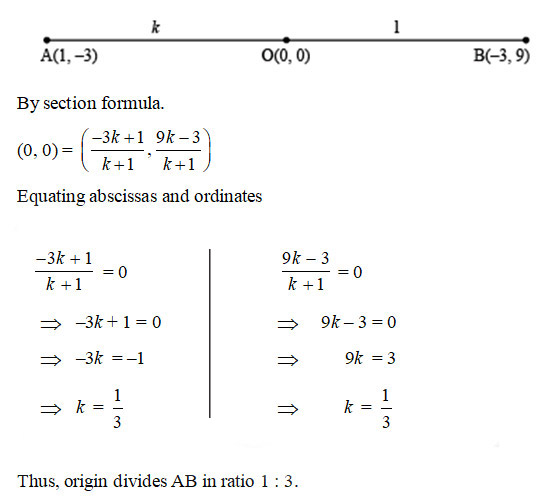## The perpendicular bisector of a line segment A(–8, 0) and B(8, 0) passes through a point (0, k). The value of k is

1. 0 only
2. 0 or 8 only
3. any real number
4. any non-zero real number

Sol. (c); The coordinates of mid-point of line segment joining A(–8, 0) and B(8, 0) =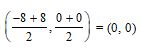Since, given that perpendicular bisector AB passes through (0, k).

Thus, the value of k will be any real number as origin is the mid-point of AB.

## Which of the following is a correct statement?

1. Two congruent figures are always similar.
2. Two similar figures are always congruent.
3. All rectangles are similar.
4. The polygons having same number of sides are similar.

Sol. (a);

1. Two congruent figures are always similar.
2. Two similar figures are not always congruent.
3. All rectangles are not similar.
4. The polygons having same number of sides are not similar.
Thus, the correct statement is ‘Two congruent figures are always similar.’

## The solution of the pair of linear equations x = –5 and y = 6 is

1. (–5, 6)
2. (–5, 0)
3. (0, 6)
4. (0, 0)

Sol. (a); The graph of linear equations x = –5 and y = 6, intersect each other at (–5, 6).

Thus, the solution of the pair of linear equations x = –5 and y = 6 is (–5, 6).

## A circle of radius 3 units is centered at (0, 0). Which of the following points lie outside the circle?

1. (–1, –1)
2. (0, 3)
3. (1, 2)
4. (3, 1)

Sol. (d); Radius of circle = 3 units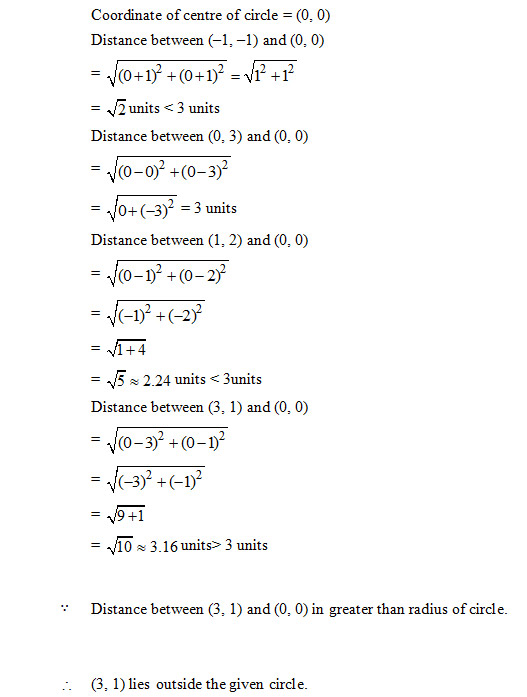## The value of k for which the pair of linear equation 3x + 5y = 8 and kx + 15y = 24 has infinitely many solutions, is

1. 3
2. 9
3. 5
4. 15

Sol. (b); The given pair of linear equations are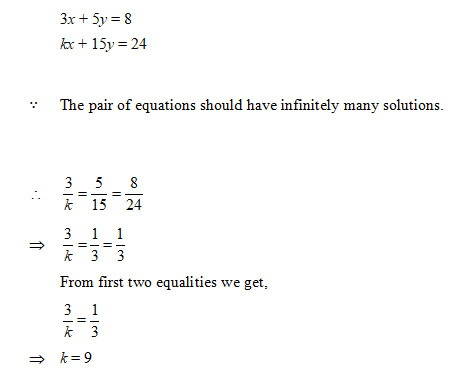## HCF of two consecutive even number is

1. 0
2. 1
3. 2
4. 4

Sol. (c); HCF of two consecutive even numbers is 2.

## The zeroes of quadratic polynomial x2 + 99x + 127 are

1. both negative
2. both positive
3. one positive and one negative
4. reciprocal of each other

Sol. (a); Let $\mathrm{\alpha }$ and β zeroes of x2 + 99x + 127.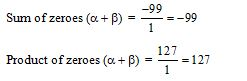Since, sum of zeroes is negative but product of zeroes is positive.

Thus, both the zeroes of the given quadratic polynomial are negative.

## The mid-point of line segment joining the points (–3, 9) and (–6, –4) is

1.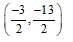2.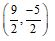3.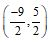4.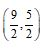Sol. (c); The coordinates of mid-point of line segment joining the points (–3, 9) and (–6, –4)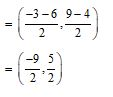#### Question 36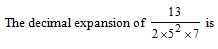1. terminating after 1 decimal place.
2. non-terminating and non-repeating.
3. terminating after 2 decimal places.
4. non-terminating but repeating.

(d); A rational number having denominator, whose prime factors are not in the form 2m × 5n, have non-terminating but repeating decimal expansion.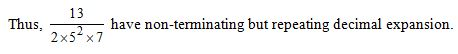## In ΔABC, DE ‖ BC, AD = 2 cm, DB = 3 cm, DE : BC is equal to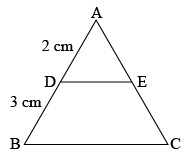1. 2 : 3
2. 2 : 5
3. 1 : 2
4. 3 : 5

Sol. (b);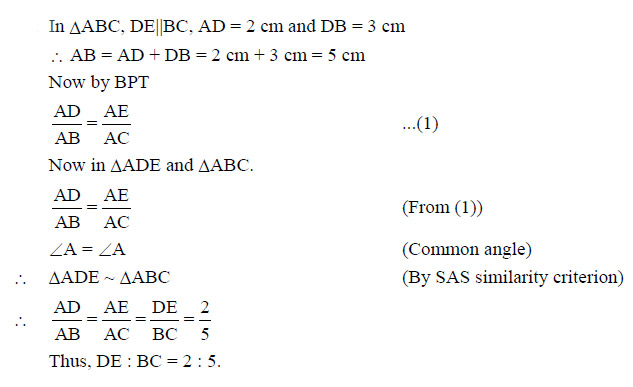## The (HCF x LCM) for the numbers 50 and 20 is

1. 1000
2. 50
3. 100
4. 500

Sol. (a); We know that,

Product of two numbers is equal to the product of their HCF and LCM.

Thus, HCF x LCM = 50 x 20 = 1000.

## For which natural number n, 6n ends with digit zero?

1. 6
2. 5
3. 0
4. None

Sol. (d);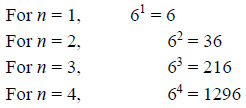We observe that for any natural number n, 6n always ends with 6.

Thus, for no natural number n, 6n ends with digit zero.

## (1 + tan2 A) (1 + sin A) (1 – sin A) is equal to

1.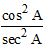2. 1
3. 0
4. 2

Sol. (b); (1 + tan2 A) (1 + sin A) (1 – sin A)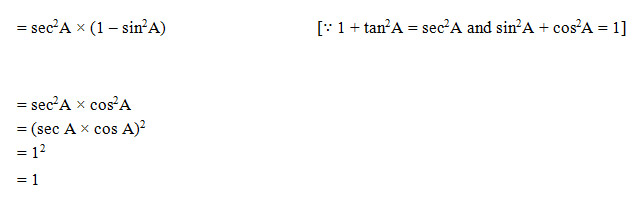### Case Study – I

(Attempt any 4 questions from Q. No. 41 to 45)

Sukriti throws a ball upwards, from a rooftop which is 8 m high from ground level. The ball reaches to some maximum height and then returns and hit the ground.

If height of the ball at time t ( in sec) is represented by h (m), then equation of its path is given as
Based above information, answer the following: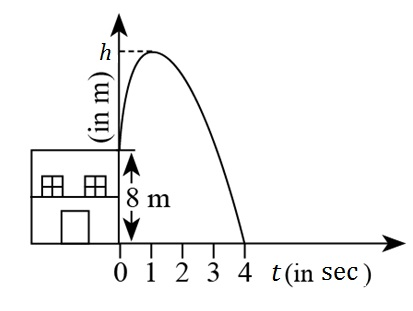## The maximum height achieved by ball is

1. 7 m
2. 8 m
3. 9 m
4. 10 m

Sol. (c)

Draw a perpendicular from maximum height on x-axis. It meet x-axis at (1, 0).

Thus, height of ball at time t = 1 is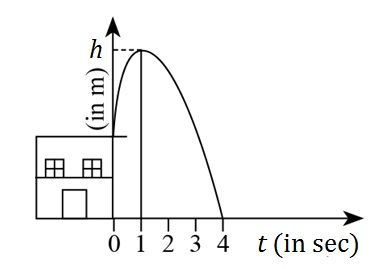## The polynomial represented by above graph is

1. linear polynomial
3. constant polynomial
4. cubic polynomial

Sol. (b) The polynomial represented by above graph is quadratic polynomial.

## Time taken by ball to reach maximum height is

1. 2 sec
2. 4 sec.
3. 1 sec.
4. 2 min.

Sol. (c); Time taken by ball to reach maximum height is 1 sec.

## Number of zeroes of the polynomial whose graph is given, is

1. 1
2. 2
3. 0
4. 3

Sol. (b) Since, given graph is of quadratic polynomial.

Thus, it has two zeros.

## Zeroes of the polynomial are

1. 4
2. –2, 4
3. 2, 4
4. 0, 4

Sol. (b); Given polynomial,

On equating to zero.

Thus, zeroes of polynomial are –2, 4.

#### Case Study- II

(Attempt any 4 questions from Q. No. 46 to 50)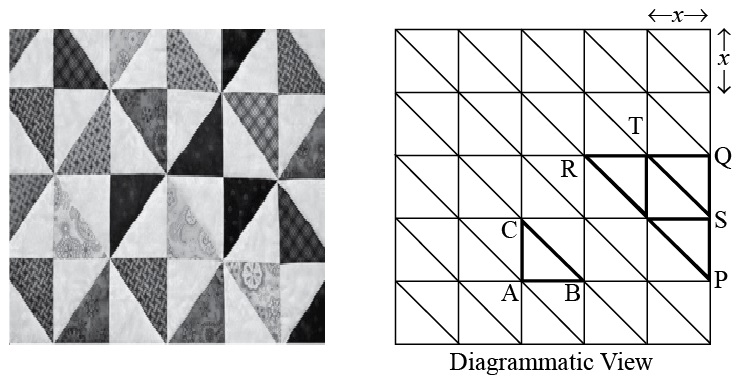Quilts are available in various colours and design. Geometric design include shapes like squares, triangles, rectangles, hexagons etc. One such design shown above. Two triangles are highlighted, .

Based on above information, answer the following questions:

## Which of the following criteria is not suitable for $△\mathrm{ABC}$ to be similar to $△\mathrm{QRP}$?

1. SAS
2. AAA
3. SSS
4. RHS

Sol. (d); RHS is not suitable for $△\mathrm{ABC}$ to be similar to $△\mathrm{QRP}$.

## If each square is of length x unit, then length BC is equal to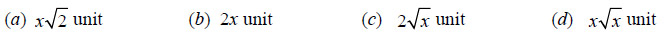Sol. (a) In right

## Ratio BC : PR is equal to

1. 2 : 1
2. 1 : 4
3. 1 : 2
4. 4 : 1

Sol. (c);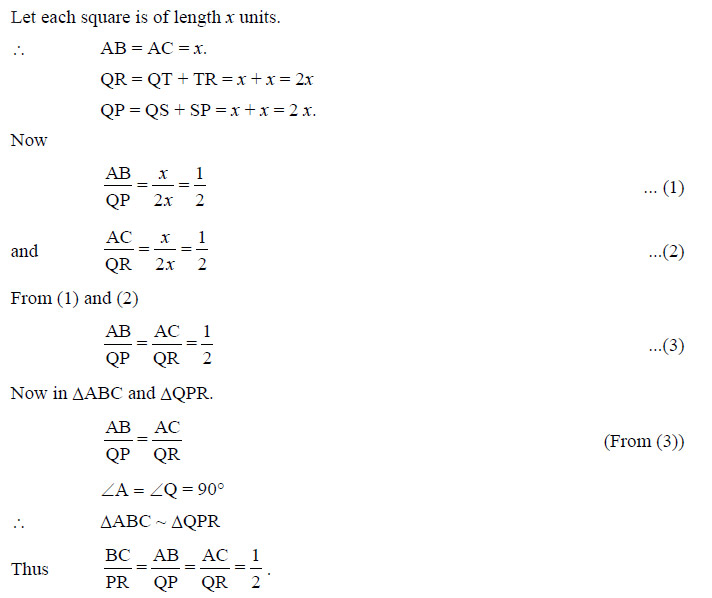## ar (PQR) : ar (ABC) is equal to

1. 2 : 1
2. 1 : 4
3. 4 : 1
4. 1 : 8

Sol. (c);

We know that, ratio of area of two similar triangles is equal to the ratio of square of corresponding sides.
Thus

Sol. (d);

It should be